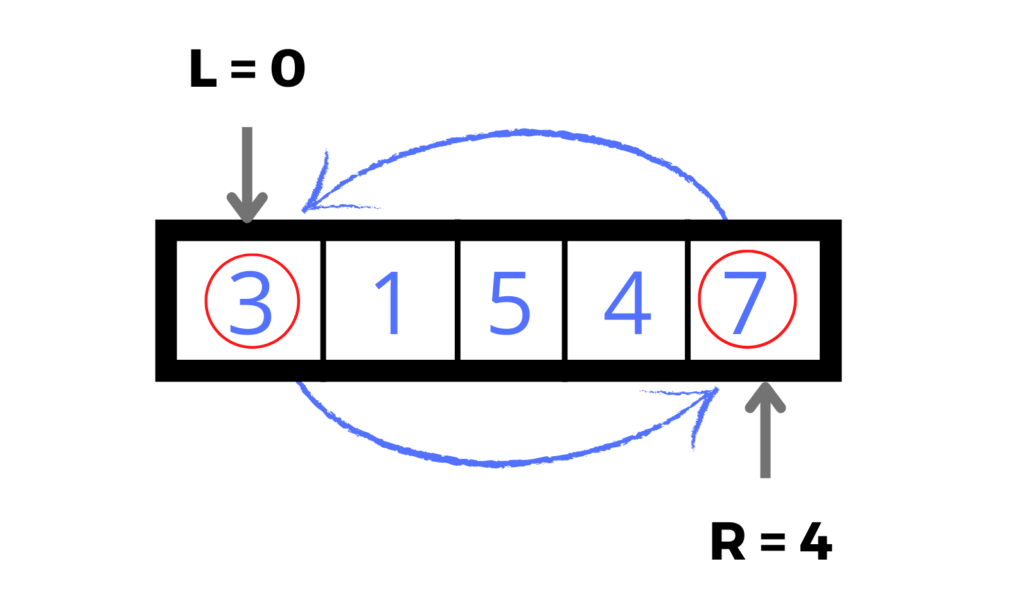×

Search anything:

# Reverse List in C++ STL [4 methods]

#### C++In this article, we have explored 4 different ways to reverse a list in C++ Standard Template Library (STL). We have explored iterative and recursive approach using Two Pointer approach as well.

1. What is STL list?
2. Method 1: Using std::list::reverse()
3. Method 2: Using std::reverse()
4. Method 3, 4: Using custom defined function (Two pointer algorithm) (recursive + iterative)

## What is STL list?

A list in STL is a sequence container that holds data of the same type. Lists are implemented as doubly linked lists(reference both the forward and backward nodes) and allow non-contiguous memory allocation.

However the implementation of lists allows constant time O(1) insertion and deletion and whereas making look up a linear operation O(n).

In this article we shall look at the different ways to reverse STL list:

• Method 1: Using std::list::reverse()
• Method 2: Using std::reverse()
• Method 3, 4: Using custom defined function (Two pointer algorithm) (recursive + iterative)

## Method 1: Using std::list::reverse()

std::list::reverse() is a void member function that reverses a list in place. It has a time complexity of O(N).

``````#include <bits/stdc++.h>
using namespace std;

int main() {
list<int> my_list = {1, 2, 3, 5, 6};

cout << "List before applying reverse(): ";
for (auto i = my_list.begin(); i != my_list.end(); i++)
cout << *i << " ";
cout << endl;

my_list.reverse();
cout << "List after appyling reverse() : ";
for (auto i = my_list.begin(); i != my_list.end(); i++)
cout << *i << " ";
return 0;
}
``````

### Output

``````List before applying reverse(): 1 2 3 5 6
List after appyling reverse() : 6 5 3 2 1
``````

## Method 2: Using std::reverse()

``````void reverse (BidirectionalIterator first, BidirectionalIterator last);
``````

std::reverse is provides a more granular approach for reversing lists. It allows reversing a given range(It might be the whole list or just a portion of the list). Similar to std::list::reverse(), it also reverses in place.

``````#include <bits/stdc++.h>
using namespace std;

int main() {
list<string> list_of_strings = { "is", "of", "the", "Hi", "Hello", "from" };
cout << "List before applying reverse(): ";
for (auto i = list_of_strings.begin(); i != list_of_strings.end(); i++) {
cout << *i << " ";
}
cout << endl;

reverse(list_of_strings.begin(), list_of_strings.end());

cout << "List after reversing: ";
for(auto i = list_of_strings.begin(); i != list_of_strings.end(); i++) {
cout << *i << " ";
}
return 0;
}
``````

### Output

``````List before applying reverse(): is of the Hi Hello from
List after reversing: from Hello Hi the of is
``````

## Method 3, 4: Using custom defined function (Two pointer algorithm) (recursive + iterative)

### Two pointer algorithmThe algorithm uses two pointers i.e first and last which initially point to the first and last elements of the list. As we traverse the list forward by incrementing the first pointer by one, we also traverse the list backwards by decrementing the last pointer by one and then swap the elements at those locations i.e the values to which the first and last pointer point are swapped.

The swapping stops when the first and last pointer overlaps

### Iterative approach

Following is the iterative C++ implementation using the above two pointer approach to reverse a list:

``````#include <bits/stdc++.h>

using namespace std;

template <class BidirectionalIterator>
void iterative_reverse(BidirectionalIterator first, BidirectionalIterator last) {
for(; first != last;) {
iter_swap(first, --last);
++first;
}
}

template <class BidirectionalIterator>
void recursive_reverse(BidirectionalIterator first,BidirectionalIterator last) {
if(first == last) {
return;
}
iter_swap(first, --last);
return recursive_reverse(++first, last);
}

int main() {
list<string> list_of_strings = { "is", "of", "the", "Hi", "Hello", "Hey" };

cout << "List before applying reverse(): ";
for (list<string>::iterator i = list_of_strings.begin(); i != list_of_strings.end(); i++) {
cout << *i << " ";
}
cout << endl;

iterative_reverse(list_of_strings.begin(), list_of_strings.end());

cout << "List after reversing: ";
for(list<string>::iterator i = list_of_strings.begin(); i != list_of_strings.end(); i++) {
cout << *i << " ";
}
return 0;
}
``````

#### Output

``````List before applying reverse(): is of the Hi Hello Hey
List after reversing: Hey Hello Hi the of is
``````

### Recursive approach

Following is the recursive C++ implementation using the above two pointer approach to reverse a list:

``````#include <bits/stdc++.h>

using namespace std;

template <class BidirectionalIterator>
void recursive_reverse(BidirectionalIterator first,BidirectionalIterator last) {
if(first == last) {
return;
}
iter_swap(first, --last);
return recursive_reverse(++first, last);
}

int main() {
list<string> list_of_strings = { "is", "of", "the", "Hi", "Hello", "Hey" };

cout << "List before applying reverse(): ";
for (list<string>::iterator i = list_of_strings.begin(); i != list_of_strings.end(); i++) {
cout << *i << " ";
}
cout << endl;

recursive_reverse(list_of_strings.begin(), list_of_strings.end());

cout << "List after reversing: ";
for(list<string>::iterator i = list_of_strings.begin(); i != list_of_strings.end(); i++) {
cout << *i << " ";
}
return 0;
}
``````

#### Output

``````List before applying reverse(): is of the Hi Hello Hey
List after reversing: Hey Hello Hi the of is
``````

With this article at OpenGenus, you must have a strong idea of how to reverse a list in C++ STL.

Happy learning#### Kirabo Ibrahim

BSTE Student in Computer Science at Makerere University, Uganda.

Reverse List in C++ STL [4 methods]